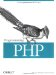5.6 Converting Between Arrays and Variables

PHP provides two functions, extract( ) and compact( ), that convert between arrays and variables. The names of the variables correspond to keys in the array, and the values of the variables become the values in the array. For instance, this array:

\$person = array('name' => 'Fred', 'age' => 35, 'wife' => 'Betty');

can be converted to, or built from, these variables:

\$name = 'Fred'; \$age  = 35; \$wife = 'Betty';

5.6.1 Creating Variables from an Array

The extract( ) function automatically creates local variables from an array. The indexes of the array elements are the variable names:

extract(\$person);                // \$name, \$age, and \$wife are now set

If a variable created by the extraction has the same name as an existing one, the extracted variable overwrites the existing variable.

You can modify extract( )'s behavior by passing a second argument. Appendix A describes the possible values for this second argument. The most useful value is EXTR_PREFIX_SAME, which says that the third argument to extract( ) is a prefix for the variable names that are created. This helps ensure that you create unique variable names when you use extract( ). It is good PHP style to always use EXTR_PREFIX_SAME, as shown here:

\$shape = "round"; \$array = array("cover" => "bird", "shape" => "rectangular"); extract(\$array, EXTR_PREFIX_SAME, "book"); echo "Cover: \$book_cover, Book Shape: \$book_shape, Shape: \$shape"; Cover: bird, Book Shape: rectangular, Shape: round

5.6.2 Creating an Array from Variables

The compact( ) function is the complement of extract( ). Pass it the variable names to compact either as separate parameters or in an array. The compact( ) function creates an associative array whose keys are the variable names and whose values are the variable's values. Any names in the array that do not correspond to actual variables are skipped. Here's an example of compact( ) in action:

\$color = 'indigo'; \$shape = 'curvy'; \$floppy = 'none'; \$a = compact('color', 'shape', 'floppy'); // or \$names = array('color', 'shape', 'floppy'); \$a = compact(\$names);Programming PHP
ISBN: 1565926102
EAN: 2147483647
Year: 2007
Pages: 168

Similar book on Amazon